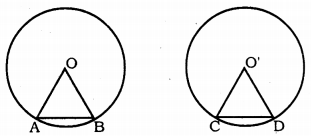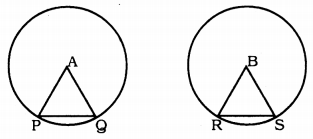KSEEB Solutions for Class 9 Maths Chapter 12 Circles Ex 12.2

Karnataka Board Class 9 Maths Chapter 12 Circles Ex 12.2

Question 1.
Recall that two circles are congruent if they have the same radii. Prove that equal chords of congruent circles subtend equal angles at their centres.
Solution:Data: Two circles having centres O and O’ and having equal radius. Chord AB = CD.
To Prove: Equal chords of congruent circles subtend equal angles at their centres.
Or ∠AOB = ∠CO’D
Proof: In ∆AOB and ∆CO’D,
OA = O’C (Data)
OB = O’D (Data)
AB = CD (Data)
∴ ∆AOB ≅ ∆CO’D
∴ ∠AOB = ∠CO’D.

Question 2.
Prove that if chords or congruent circles subtend equal angles at their centres, then the chords are equal.
Solution:Circle with centres A and B are congruent and ∠PAQ = ∠RBS
To Prove PQ = RS.
Proof: In ∆APQ and ∆BRS,
AP = BR ……… radii of congruent circles
AQ = BS are equal
∠PAQ = ∠RBS (Data)
∴ ∆PQR ≅ ∆BRS (SAS postulate)
∴ PQ = RS.